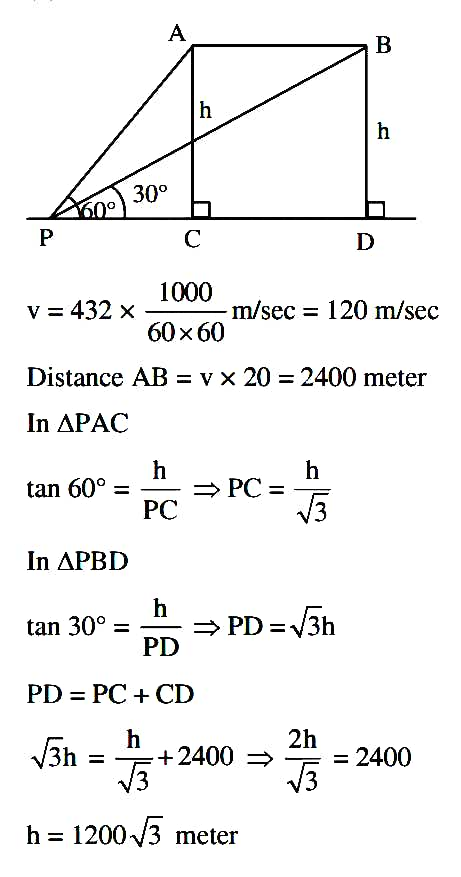# A aeroplane is flying horizontally with speed of 432 km/hr at height h meter from ground. Its angle of elevation

more_vert

A aeroplane is flying horizontally with speed of 432 km/hr at height h meter from ground. Its angle of elevation from a point on ground is 60°. After 20 sec its angle of elevation from same point is 30° then the height 'h' is equal to

$$A) 1200\sqrt3$$

$$B) 600\sqrt3$$

$$C) 1800\sqrt3$$

$$D) 1000\sqrt3$$

more_vert

verified

The correct answer is option A)1200$$\sqrt3$$

Explanation::